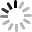# SVG D3.js - 定義比例 ( scale.linear() )## 定義比例Quantitative Scale 又分為 linear、pow、log、quantize、threshold、quantile 和 identity，其中又以 linear 最常使用，這篇也將主要介紹 scale.linear() 的用法;scale.linear() 具有以下幾個 API 可以使用：

• linear.domain([numbers])
• linear.range([values])
• linear.invert(y)
• linear.rangeRound(values)
• linear.clamp([boolean])
• linear.nice([count])
• linear.interpolate([factory])
• linear.ticks([count])
• linear.tickFormat(count, [format])
• linear.copy()

## linear.domain([numbers])、linear.range([values])``````  var data = [
{x:0, y:1.89},
{x:1, y:2.77},
{x:2, y:0.86},
{x:3, y:3.45},
{x:4, y:4.13},
{x:5, y:3.59},
{x:6, y:2.33},
{x:7, y:3.79},
{x:8, y:2.61},
{x:9, y:2.15}
];

var width = 240,
height = 120;

var s = d3.select('#s');

s.attr({
'width': width,
'height': height,
}).style({
'border':'1px solid #000'
});

var line = d3.svg.line()
.x(function(d) {
return d.x;
}).y(function(d) {
return d.y;
});

s.append('path')
.attr({
'd':line(data),
'stroke':'#09c',
'fill':'none'
});
</script>
````````````  var scaleX = d3.scale.linear()
.range([0,width])
.domain([0,9]);

var scaleY = d3.scale.linear()
.range([0,height])
.domain([0,5]);

var line = d3.svg.line()
.x(function(d) {
return scaleX(d.x);
}).y(function(d) {
return scaleY(d.y);
});
````````````  var data = [
{x:0, y:100},
{x:10, y:154},
{x:20, y:288},
{x:30, y:187},
{x:40, y:235},
{x:50, y:198},
{x:60, y:172},
{x:70, y:134},
{x:80, y:94},
{x:90, y:88}
];
````````````  var scaleX = d3.scale.linear()
.range([0,width])
.domain([0,90]);

var scaleY = d3.scale.linear()
.range([0,height])
.domain([0,300]);

var line = d3.svg.line()
.x(function(d) {
return scaleX(d.x);
}).y(function(d) {
return scaleY(d.y);
});
````````````  var data = [
{x:0, y:1.89},
{x:1, y:2.77},
{x:2, y:0.86},
{x:3, y:3.45},
{x:4, y:4.13},
{x:5, y:3.59},
{x:6, y:2.33},
{x:7, y:3.79},
{x:8, y:2.61},
{x:9, y:2.15}
];

var width = 240,
height = 120;

var s = d3.select('#s');

s.attr({
'width': width,
'height': height,
}).style({
'border':'1px solid #000'
});

var minX = d3.min(data, function(d){return d.x});
var maxX = d3.max(data, function(d){return d.x});
var minY = d3.min(data, function(d){return d.y});
var maxY = d3.max(data, function(d){return d.y});

var scaleX = d3.scale.linear()
.range([0,width])
.domain([minX,maxX]); //x 的最大值與最小值

var scaleY = d3.scale.linear()
.range([height,0])    //反過來才會上下顛倒
.domain([minY,maxY]); //y 的最大值與最小值

var line = d3.svg.line()
.x(function(d) {
return scaleX(d.x);
}).y(function(d) {
return scaleY(d.y);
});

s.append('path')
.attr({
'd':line(data),
'stroke':'#09c',
'fill':'none'
});
``````## linear.clamp([boolean])

clamp 的內容是放 true 或 false 的布林值，這只是一個開關的設定，預設是 flase，為什麼說是開關呢？因為當我們沒有設定或設為 flase 的時候，超過 domain 最大值的數字將仍然按照 range 的比例進行縮放，但若設定為 true，超過最大值的數字一律以最大值呈現。``````  var scaleX = d3.scale.linear()
.range([0, width])
.domain([0, 9])
.clamp(true);
``````## linear.nice([count])

nice 會根據整體 range 的狀況，改變函數的 domain，使 domain 內的範圍值返回最接近的數，例如 0.986743 就返回 1.0，0.444 就返回 0.45，範例使用一個有套用 nice 一個沒有，就可以很明顯的看出差異，如果把 console 打開來看，就可以發現套用 nice 的數值明顯變了許多。( 範例：svg-d3-03-scale-linear-demo8.html )

``````  //對照組，沒有使用 .nice()
var scaleX1 = d3.scale.linear()
.range([0, width])
.domain([0.123, 9.189]);

var scaleY1 = d3.scale.linear()
.range([height, 0])
.domain([0.123, 5.567]);

var line1 = d3.svg.line()
.x(function(d) {
return scaleX1(d.x);
}).y(function(d) {
return scaleY1(d.y);
});

//實驗組，使用 .nice()
var scaleX2 = d3.scale.linear()
.range([0, width])
.domain([0.123, 9.189]).nice();

var scaleY2 = d3.scale.linear()
.range([height, 0])
.domain([0.123, 5.567]).nice();

var line2 = d3.svg.line()
.x(function(d) {
return scaleX2(d.x);
}).y(function(d) {
return scaleY2(d.y);
});

s.append('path')
.attr({
'd': line1(data),
'stroke': '#f66',
'fill': 'none'
});

s.append('path')
.attr({
'd': line2(data),
'stroke': '#09c',
'fill': 'none'
});
``````## linear.rangeRound(values)

``````  //對照組，range()
var scaleX1 = d3.scale.linear()
.range([0, width])
.domain([0, 9]);

var scaleY1 = d3.scale.linear()
.range([120.967, 0.678])
.domain([0, 5]);

var line1 = d3.svg.line()
.x(function(d) {
return scaleX1(d.x);
}).y(function(d) {
return scaleY1(d.y);
});

//實驗組，rangeRound()
var scaleX2 = d3.scale.linear()
.rangeRound([0, width])
.domain([0, 9]);

var scaleY2 = d3.scale.linear()
.rangeRound([120.967, 0.678])
.domain([0, 5]);

var line2 = d3.svg.line()
.x(function(d) {
return scaleX2(d.x);
}).y(function(d) {
return scaleY2(d.y);
});
``````## linear.invert(y)

``````console.log(scaleX(2.5));             //得到 66.66666666666667
console.log(scaleX.invert(66.6667));  //得到 2.50000125
``````## linear.ticks([count])、linear.tickFormat(count, [format])

ticks 和 tickFormat 通常都會一起看，ticks 會根據內容數值的範圍，按照 count 的數量來做切割，取出最適當的數值區間，預設值為 10，不過若按照數字切割出來的範圍不適當，有時不一定會按照我們所設的數字切割範圍，而 tickFormat 就是可以設定數值的格式，這兩者在 Axis 座標裏頭比較有用，這裡可以使用 console 看出差異。( 範例：svg-d3-03-scale-linear-demo11.html )

``````console.log(
scaleX.ticks(10)
);
console.log(
scaleX.ticks(10).map(scaleX.tickFormat(1,"%"))
);
//格式可以參考 https://github.com/mbostock/d3/wiki/Formatting#d3_format
``````## linear.copy()

copy 很容易理解，就單純是複製一份 range ，而不會影響到原本的 range。( 範例：svg-d3-03-scale-linear-demo12.html )

``````   var scaleX2 = scaleX1.copy();

var scaleY2 = d3.scale.linear()
.range([height, 0])
.domain([0, 10]);

var line2 = d3.svg.line()
.x(function(d) {
return scaleX2(d.x);
}).y(function(d) {
return scaleY2(d.y);
});
``````## linear.interpolate([factory])

• d3.interpolateNumber
• d3.interpolateRound
• d3.interpolateString
• d3.interpolateRgb
• d3.interpolateHsl
• d3.interpolateLab
• d3.interpolateHcl
• d3.interpolateArray
• d3.interpolateObject
• d3.interpolateTransform
• d3.interpolateZoom
• d3.interpolators

## 小結

• Identity: linear 的特例，主要針對 1:1 的縮放。
• Power and Logarithmic scales: 利用指數函數來進行比例縮放。
• Quantize and Quantile scales: 離散並量化的比例縮放。